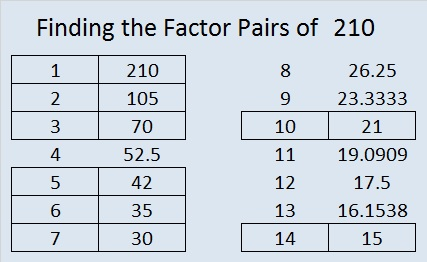# 210 and Level 4

• 210 is a composite number and a triangular number.
• Prime factorization: 210 = 2 x 3 x 5 x 7, so 210 is the product of the first four prime numbers!
• The exponents in the prime factorization are 1, 1, 1, and 1. Adding one to each and multiplying we get (1 + 1)(1 + 1)(1 + 1)(1 + 1) = 2 x 2 x 2 x 2 = 16. Therefore 210 has 16 factors.
• Factors of 210: 1, 2, 3, 5, 6, 7, 10, 14, 15, 21, 30, 35, 42, 70, 105, 210
• Factor pairs: 210 = 1 x 210, 2 x 105, 3 x 70, 5 x 42, 6 x 35, 7 x 30, 10 x 21, or 14 x 15
• Since 210 has no square factors, √210 cannot be simplified. √210 ≈ 14.491Because 20 + 1 = 21 and (20/2) x 21 = 210, we know that 1 + 2 + 3 + 4 + 5 + 6 + 7 + 8 + 9 + 10 + 11 + 12 + 13 + 14 + 15 + 16 + 17 + 18 + 19 + 20 = 210, thus it is the 20th triangular number.

Excel file of puzzles and previous week’s factor solutions: 10 Factors 2014-08-18This site uses Akismet to reduce spam. Learn how your comment data is processed.